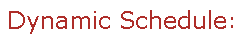Visit Minerva Class Schedule for course dates & times.

# MATH 236 Algebra 2 (3 unités)

Offered by: Math. et statistique (Sciences)

### Vue d'ensemble

Mathématiques et Statistiques (Sci) : Linear equations over a field. Introduction to vector spaces. Linear mappings. Matrix representation of linear mappings. Determinants. Eigenvectors and eigenvalues. Diagonalizable operators. Cayley-Hamilton theorem. Bilinear and quadratic forms. Inner product spaces, orthogonal diagonalization of symmetric matrices. Canonical forms.

Terms: Hiver 2023

Instructors: Sroka, Marcin (Winter)